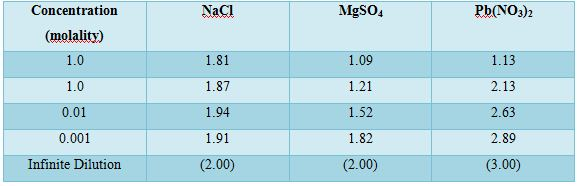# Colligative Properties from Dissociation in Solution

Abnormal Molecular Masses from Colligative Properties from Dissociation in solution

The laws of the colligative properties are applicable only for very dilute solution, and when there are no association and dissociation of the solutes in solution. Sometimes abnormal molecular masses of the solutes were observed when calculated from the laws of colligative properties. Let us consider the association and dissociation of solutes in solutions.

Dissociation in solution: Van’t Hoff pointed out that dilute aqueous solutions of inorganic salts, acids and bases give abnormally large osmotic pressure. He could not account for the anomaly but found that the introduction of an empirical factor, i, in the osmotic pressure equation gave agreement with experimental values. This equation then becomes,

πobs = i CRT

Such anomaly was also observed in the case of freezing point depression, boiling point elevation and lowering of vapour pressure and ‘i’ could be expressed, in the form,

i = π/π0 = ∆p/∆p0 = ∆T/∆Tf

where the numerators are the experimental values and the quantities in the denominator are the values expected from the respective equation.

At first ‘i’ was regarded at a constant, but subsequent investigation showed that the value increases with the decrease of concentration of the solute. The values of ‘i’ for several Salt in water are given in table.

Table: Van’t Hoff factor for several salts in eater obtained from measurements of freezing point depressionThe abnormally high values of the colligative properties of salts, acids and bases in aqueous solution were explained by Arrhenius who proposed that in aqueous solutions a certain fraction of the molecules of acids, bases and salts dissociate to form positively and negatively charged ions. As a result the number of discrete units in solution increases giving abnormally large colligative properties of such solutions. He proposed that the degree of dissociation increases with the decrease of concentration. This explains why the value of the Van’t Hoff factor increases as the concentration is decreased. On the basis of a postulate that equilibrium exists between the dissociated and undissociated molecules Arrhenius was able to calculate the degree of dissociation of the electrolytes from osmotic pressure, freezing point depression and boiling point elevation of solutions.